# Resources tagged with: Mathematical modelling

Filter by: Content type:
Age range:
Challenge level:

### There are 67 results

Broad Topics > Thinking Mathematically > Mathematical modelling### Population Dynamics - Part 1

##### Age 16 to 18 Challenge Level:

First in our series of problems on population dynamics for advanced students.### Population Dynamics - Part 6

##### Age 16 to 18 Challenge Level:

Sixth in our series of problems on population dynamics for advanced students.### Population Dynamics - Part 2

##### Age 16 to 18 Challenge Level:

Second in our series of problems on population dynamics for advanced students.### Population Dynamics - Part 3

##### Age 16 to 18 Challenge Level:

Third in our series of problems on population dynamics for advanced students.### Population Dynamics - Part 4

##### Age 16 to 18 Challenge Level:

Fourth in our series of problems on population dynamics for advanced students.### Population Dynamics - Part 5

##### Age 16 to 18 Challenge Level:

Fifth in our series of problems on population dynamics for advanced students.### Population Dynamics Collection

##### Age 16 to 18 Challenge Level:

This is our collection of tasks on the mathematical theme of 'Population Dynamics' for advanced students and those interested in mathematical modelling.### Population Ecology Using Probability

##### Age 16 to 18 Challenge Level:

An advanced mathematical exploration supporting our series of articles on population dynamics for advanced students.### Bird-brained

##### Age 16 to 18 Challenge Level:

How many eggs should a bird lay to maximise the number of chicks that will hatch? An introduction to optimisation.### Pdf Stories

##### Age 16 to 18 Challenge Level:

Invent scenarios which would give rise to these probability density functions.### FA Cup

##### Age 16 to 18 Challenge Level:

In four years 2001 to 2004 Arsenal have been drawn against Chelsea in the FA cup and have beaten Chelsea every time. What was the probability of this? Lots of fractions in the calculations!### Rocking Chairs, Railway Games and Rayboxes

##### Age 5 to 18

In this article for teachers, Alan Parr looks at ways that mathematics teaching and learning can start from the useful and interesting things can we do with the subject, including. . . .### Branching Processes and Extinction

##### Age 16 to 18 Challenge Level:

An advanced mathematical exploration supporting our series of articles on population dynamics for advanced students.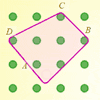### Elastic Maths

##### Age 14 to 18

How do you write a computer program that creates the illusion of stretching elastic bands between pegs of a Geoboard? The answer contains some surprising mathematics.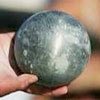### Model Solutions

##### Age 16 to 18 Challenge Level:

How do these modelling assumption affect the solutions?### The Wrong Stats

##### Age 16 to 18 Challenge Level:

Why MUST these statistical statements probably be at least a little bit wrong?### Bionrich

##### Age 14 to 18 Challenge Level:

bioNRICH is the area of the stemNRICH site devoted to the mathematics underlying the study of the biological sciences, designed to help develop the mathematics required to get the most from your. . . .### Predator - Prey Systems

##### Age 16 to 18 Challenge Level:

See how differential equations might be used to make a realistic model of a system containing predators and their prey.##### Age 16 to 18 Challenge Level:

This is the section of stemNRICH devoted to the advanced applied mathematics underlying the study of the sciences at higher levels### Population Dynamics

##### Age 16 to 18 Challenge Level:

This problem opens a major sequence of activities on the mathematics of population dynamics for advanced students.### Spinners

##### Age 16 to 18 Challenge Level:

How do scores on dice and factors of polynomials relate to each other?### Ramping it Up

##### Age 16 to 18 Challenge Level:

Look at the calculus behind the simple act of a car going over a step.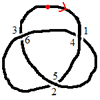### Drawing Doodles and Naming Knots

##### Age 7 to 18

This article for students introduces the idea of naming knots using numbers. You'll need some paper and something to write with handy!### Ball Bearings

##### Age 16 to 18 Challenge Level:

If a is the radius of the axle, b the radius of each ball-bearing, and c the radius of the hub, why does the number of ball bearings n determine the ratio c/a? Find a formula for c/a in terms of n.### The Mean Game

##### Age 16 to 18

Edward Wallace based his A Level Statistics Project on The Mean Game. Each picks 2 numbers. The winner is the player who picks a number closest to the mean of all the numbers picked.### Over-booking

##### Age 16 to 18 Challenge Level:

The probability that a passenger books a flight and does not turn up is 0.05. For an aeroplane with 400 seats how many tickets can be sold so that only 1% of flights are over-booked?### Guessing the Graph

##### Age 14 to 16 Challenge Level:

Can you suggest a curve to fit some experimental data? Can you work out where the data might have come from?### Cushion Ball

##### Age 16 to 18 Challenge Level:

The shortest path between any two points on a snooker table is the straight line between them but what if the ball must bounce off one wall, or 2 walls, or 3 walls?### Physnrich

##### Age 14 to 18 Challenge Level:

PhysNRICH is the area of the StemNRICH site devoted to the mathematics underlying the study of physics### Engnrich

##### Age 16 to 18 Challenge Level:

engNRICH is the area of the stemNRICH Advanced site devoted to the mathematics underlying the study of engineering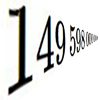### Big and Small Numbers in Physics - Group Task

##### Age 16 to 18 Challenge Level:

Work in groups to try to create the best approximations to these physical quantities.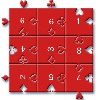### Impuzzable

##### Age 16 to 18

This is about a fiendishly difficult jigsaw and how to solve it using a computer program.### The Not-so-simple Pendulum 1

##### Age 16 to 18 Challenge Level:

See how the motion of the simple pendulum is not-so-simple after all.### Time to Evolve 2

##### Age 16 to 18 Challenge Level:

How is the length of time between the birth of an animal and the birth of its great great ... great grandparent distributed?### Maximum Flow

##### Age 16 to 18 Challenge Level:

Given the graph of a supply network and the maximum capacity for flow in each section find the maximum flow across the network.### Truth Tables and Electronic Circuits

##### Age 11 to 18

Investigate circuits and record your findings in this simple introduction to truth tables and logic.### The Use of Mathematics in Computer Games

##### Age 16 to 18

An account of how mathematics is used in computer games including geometry, vectors, transformations, 3D graphics, graph theory and simulations.### Chemnrich

##### Age 14 to 18 Challenge Level:

chemNRICH is the area of the stemNRICH site devoted to the mathematics underlying the study of chemistry, designed to help develop the mathematics required to get the most from your study. . . .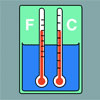### Stemnrich - the Physical World

##### Age 11 to 16 Challenge Level:

PhysNRICH is the area of the StemNRICH site devoted to the mathematics underlying the study of physics### Investigating Epidemics

##### Age 11 to 16 Challenge Level:

Simple models which help us to investigate how epidemics grow and die out.### Designing Table Mats

##### Age 11 to 16 Challenge Level:

Formulate and investigate a simple mathematical model for the design of a table mat.### In Constantly Passing

##### Age 14 to 16 Challenge Level:

A car is travelling along a dual carriageway at constant speed. Every 3 minutes a bus passes going in the opposite direction, while every 6 minutes a bus passes the car travelling in the same. . . .### An Introduction to Computer Programming and Mathematics

##### Age 16 to 18

This article explains the concepts involved in scientific mathematical computing. It will be very useful and interesting to anyone interested in computer programming or mathematics.### Modelling Assumptions in Mechanics

##### Age 16 to 18

An article demonstrating mathematically how various physical modelling assumptions affect the solution to the seemingly simple problem of the projectile.### Stonehenge

##### Age 16 to 18 Challenge Level:

Explain why, when moving heavy objects on rollers, the object moves twice as fast as the rollers. Try a similar experiment yourself.### Snooker

##### Age 16 to 18 Challenge Level:

A player has probability 0.4 of winning a single game. What is his probability of winning a 'best of 15 games' tournament?### Problem Solving, Using and Applying and Functional Mathematics

##### Age 5 to 18 Challenge Level:

Problem solving is at the heart of the NRICH site. All the problems give learners opportunities to learn, develop or use mathematical concepts and skills. Read here for more information.### Escalator

##### Age 14 to 16 Challenge Level:

At Holborn underground station there is a very long escalator. Two people are in a hurry and so climb the escalator as it is moving upwards, thus adding their speed to that of the moving steps. . . .
### Concrete Calculation

##### Age 14 to 16 Challenge Level:

The builders have dug a hole in the ground to be filled with concrete for the foundations of our garage. How many cubic metres of ready-mix concrete should the builders order to fill this hole to. . . .### Shaping the Universe III - to Infinity and Beyond

##### Age 11 to 16

The third installment in our series on the shape of astronomical systems, this article explores galaxies and the universe beyond our solar system.